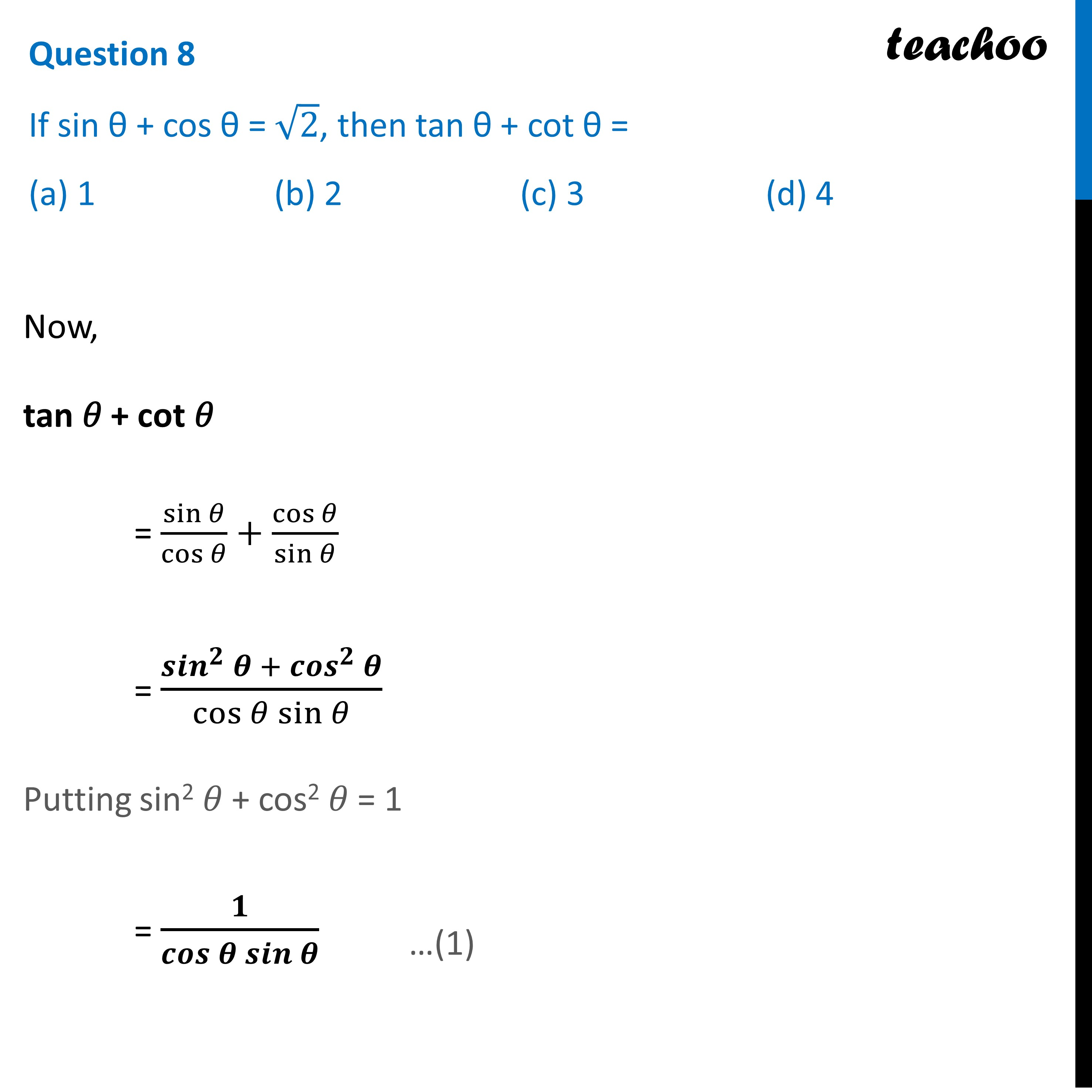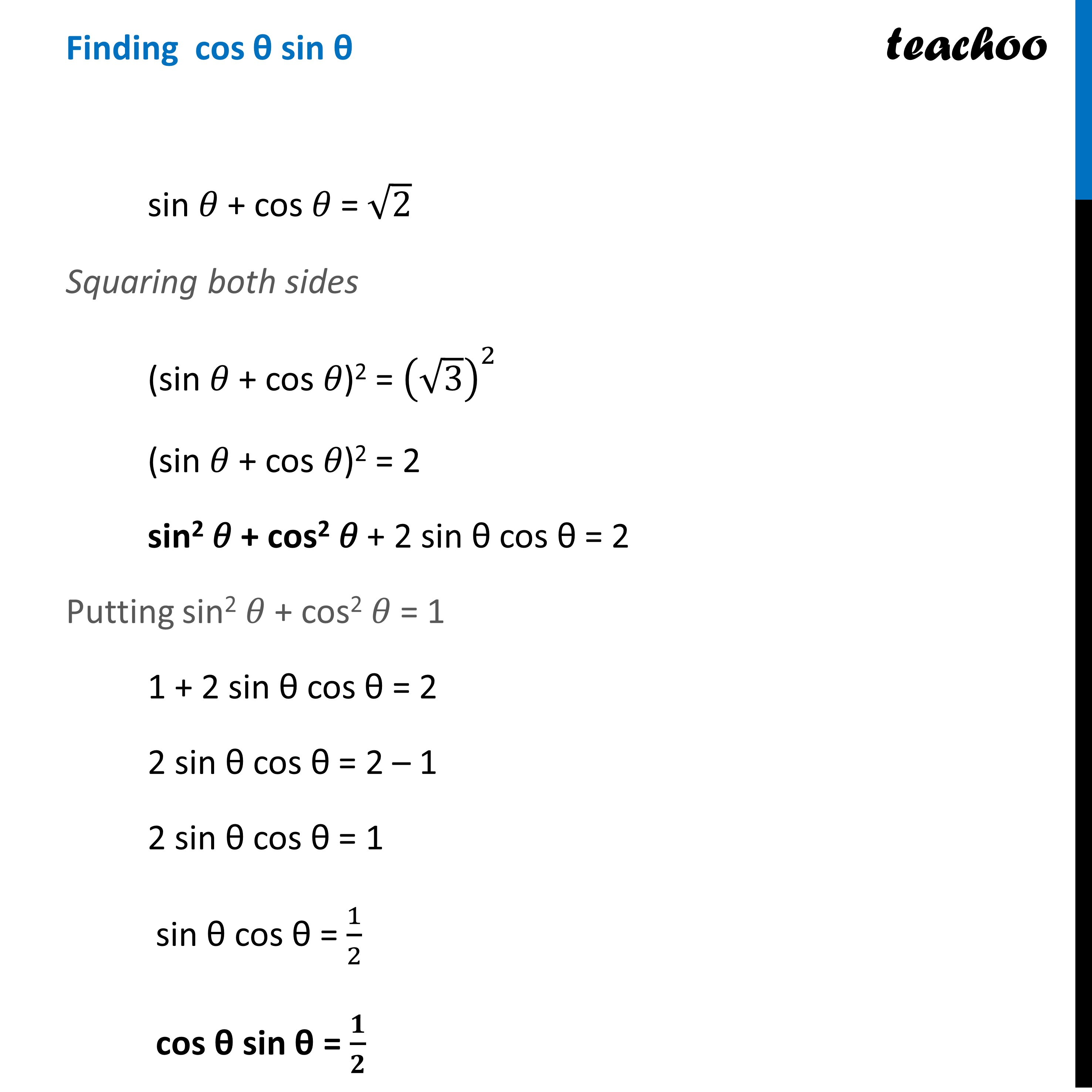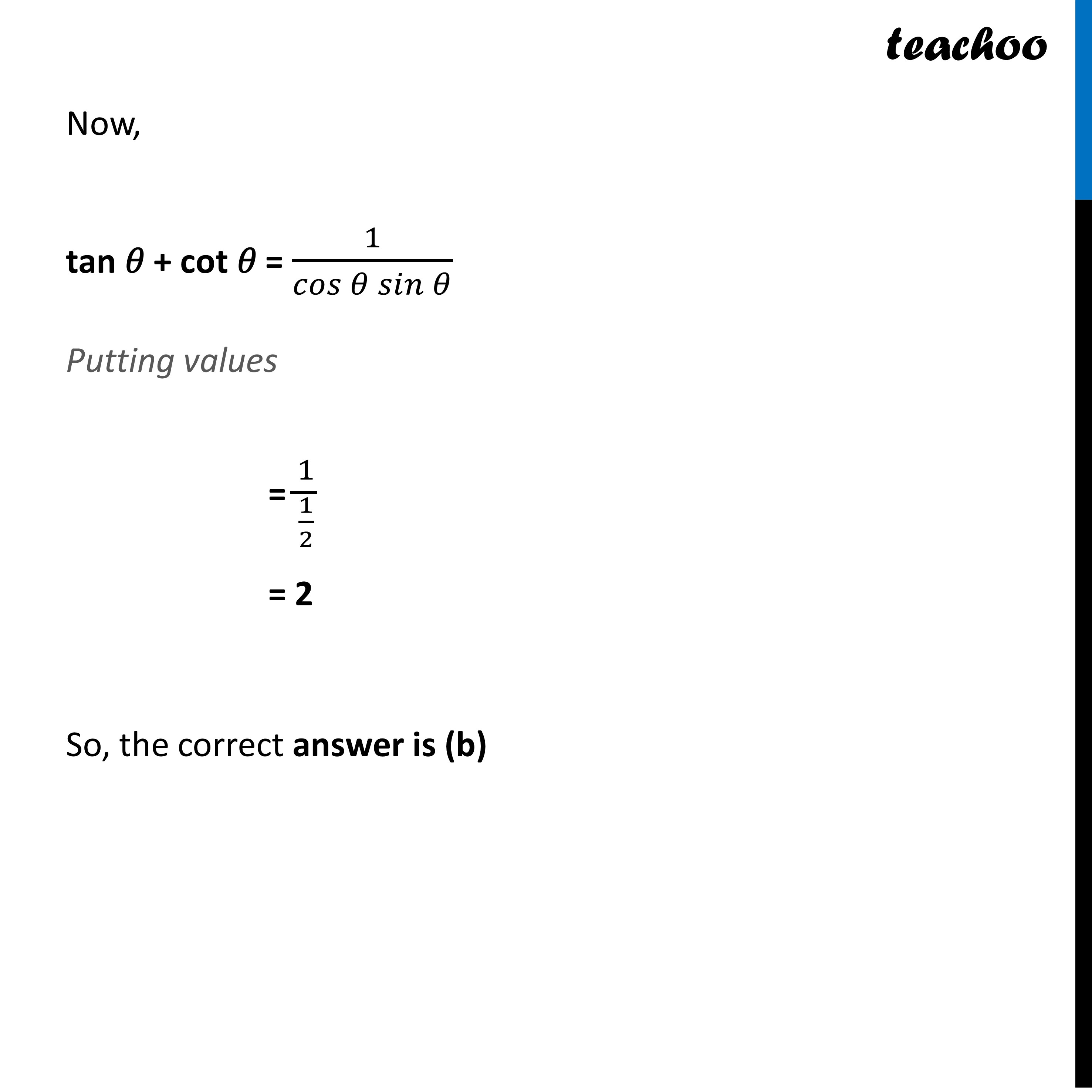CBSE Class 10 Sample Paper for 2023 Boards - Maths Standard

Class 10
Solutions of Sample Papers for Class 10 Boards

## (a) 1   (b) 2   (c) 3   (d) 4

This question is Similar to Question 8 CBSE Class 10 Sample Paper for 2020 Boards - Maths StandardGet live Maths 1-on-1 Classs - Class 6 to 12

### Transcript

Question 8 If sin θ + cos θ = √2, then tan θ + cot θ = (a) 1 (b) 2 (c) 3 (d) 4 Now, tan 𝜃 + cot 𝜃 = sin⁡𝜃/cos⁡𝜃 +cos⁡𝜃/sin⁡𝜃 = (〖𝒔𝒊𝒏〗^𝟐⁡𝜽 + 〖𝒄𝒐𝒔〗^𝟐⁡𝜽)/(cos⁡𝜃 sin⁡𝜃 ) Putting sin2 𝜃 + cos2 𝜃 = 1 = 𝟏/(𝒄𝒐𝒔⁡𝜽 𝒔𝒊𝒏⁡𝜽 ) Finding cos θ sin θ sin 𝜃 + cos 𝜃 = √2 Squaring both sides (sin 𝜃 + cos 𝜃)2 = (√3)^2 (sin 𝜃 + cos 𝜃)2 = 2 sin2 𝜃 + cos2 𝜃 + 2 sin θ cos θ = 2 Putting sin2 𝜃 + cos2 𝜃 = 1 1 + 2 sin θ cos θ = 2 2 sin θ cos θ = 2 – 1 2 sin θ cos θ = 1 sin θ cos θ = 1/2 cos θ sin θ = 𝟏/𝟐 Now, tan 𝜃 + cot 𝜃 = 1/(𝑐𝑜𝑠⁡𝜃 𝑠𝑖𝑛⁡𝜃 ) Putting values = 1/(1/2) = 2 So, the correct answer is (b)